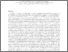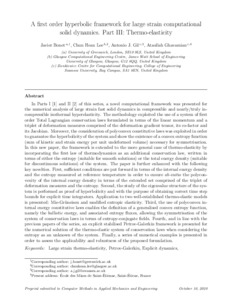A first order hyperbolic framework for large strain computational solid dynamics. Part III: Thermo-elasticity

, Lee, Chun Hean, Gil, Antonio J. and Ghavamian, Ataollah (2020) A first order hyperbolic framework for large strain computational solid dynamics. Part III: Thermo-elasticity. Computer Methods in Applied Mechanics and Engineering, 373:113505. ISSN 0045-7825 (doi:https://doi.org/10.1016/j.cma.2020.113505)Preview
PDF (Author Accepted Manuscript)
30077 BONET_A_First_Order_Hyperbolic_Framework_2020.pdf - Accepted Version

Abstract

In Parts I  and II  of this series, a novel computational framework was presented for the numerical analysis of large strain fast solid dynamics in compressible and nearly/truly incompressible isothermal hyperelasticity. The methodology exploited the use of a system of first order Total Lagrangian conservation laws formulated in terms of the linear momentum and a triplet of deformation measures comprised of the deformation gradient tensor, its co-factor and its Jacobian. Moreover, the consideration of polyconvex constitutive laws was exploited in order to guarantee the hyperbolicity of the system and show the existence of a convex entropy function (sum of kinetic and strain energy per unit undeformed volume) necessary for symmetrisation. In this new paper, the framework is extended to the more general case of thermo-elasticity by incorporating the first law of thermodynamics as an additional conservation law, written in terms of either the entropy (suitable for smooth solutions) or the total energy density (suitable for discontinuous solutions) of the system. The paper is further enhanced with the following key novelties. First, sufficient conditions are put forward in terms of the internal energy density and the entropy measured at reference temperature in order to ensure ab-initio the polyconvexity of the internal energy density in terms of the extended set comprised of the triplet of deformation measures and the entropy. Second, the study of the eigenvalue structure of the system is performed as proof of hyperbolicity and with the purpose of obtaining correct time step bounds for explicit time integrators. Application to two well-established thermo-elastic models is presented: Mie-Gruneisen and modified entropic elasticity. Third, the use of polyconvex internal energy constitutive laws enables the definition of a generalised convex entropy function, namely the ballistic energy, and associated entropy fluxes, allowing the symmetrisation of the system of conservation laws in terms of entropy-conjugate fields. Fourth, and in line with the previous papers of the series, an explicit stabilised Petrov-Galerkin framework is presented for the numerical solution of the thermo-elastic system of conservation laws when considering the entropy as an unknown of the system. Finally, a series of numerical examples is presented in order to assess the applicability and robustness of the proposed formulation.

Item Type: Article Large strain thermo-elasticity, Petrov-Galerkin, Explicit dynamics, Polyconvexity, Conservation laws, Ballistic energy T Technology > TA Engineering (General). Civil engineering (General) Vice-Chancellor's Group 03 Nov 2021 01:38 None None None None None http://gala.gre.ac.uk/id/eprint/30077View Item